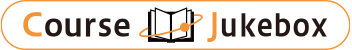### CourseDetailDegree
Master
Course delivery methods
face-to-face
Subject
Computer Science
Program
School
College of Electrical Engineering & Computer Science
Department
Campus
Main Campus
Classroom
Course Offering Year
Course Offering Month
February - June
Weekday and Period
Monday 4 Thursday 8,9
Capacity
50
Credits
3
Language
English
Course Number
EE2007 (901E21000)

### Probability and Statistics National Taiwan University

#### Course Overview

Tentative Course Outline: 1. Experiments, Models, and Probabilities 1.1. Applying Set Theory to Probability 1.2. Probability Axioms 1.3. Some Consequences of the Axioms 1.4. Conditional Probability 1.5. Independence 1.6. Sequential Experiments and Tree Diagrams 2. Random Variables 2.1. Definitions 2.2. Probability Mass Function 2.3. Families of Discrete Random Variables 2.4. Cumulative Distribution Function (CDF) 2.5. Probability Density Function 2.6. Families of Continuous Random Variables 3. Random Variables and Expected Value 3.1. Conditional Probability Mass/Density Function 3.2. Probability Models of Derived Random Variables 3.3. Average 3.4. Variance and Standard Deviation 3.5. Expected Value of a Derived Random Variable Midterm exam 4. Random Vectors 4.1. Probability Models of N Random Variables 4.2. Vector Notation 4.3. Joint Cumulative Distribution Function 4.4. Joint Probability Mass/Density Function 4.5. Marginal PMF/PDF 4.6. Functions of Two Random Variables (Jacobian Transformation) 4.7. Conditioning by a Random Variables 4.8. Bivariate Gaussian Random Variables 4.9. Correlation Matrix 5. Sums of Random Variables 5.1. Expected Values of Sums 5.2. PDF of the Sum of Two Random Variables 5.3. Moment Generating Functions 5.4. MGF of the Sum of Independent Random Variables 5.5. Random Sums of Independent Random Variables 5.6. Central Limit Theorem 5.7. Applications of the Central Limit Theorem 5.8. The Chernoff Bound 6. Parameter Estimation Using the Sample Mean 6.1. Sample Mean: Expected Value and Variance 6.2. Deviation of a Random Variable from the Expected Value 6.3. Point Estimates of Model Parameters 6.4. Confidence Intervals 7. Hypothesis Testing 7.1. Significance Testing 7.2. Binary Hypothesis Testing Final exam

#### Learning Achievement

To introduce to students the theory, models and analysis of probability and basic statistics and their applications with emphasis on electrical and computer engineering problems.

#### Course prerequisites

Calculus (A) 1 & 2
Grading: Homework : 20%, Midterm : 40%, Final : 40%, Participation 5%

Shi Chung Chang

#### Other information

(College of Electrical Engineering and Computer Science) Department of Electrical Engineering